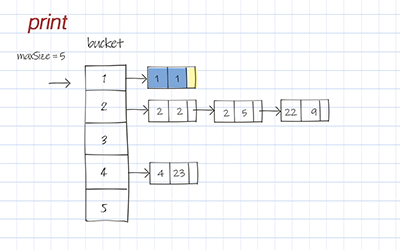Hash table is a data structure that can map keys to values. A hash table uses a hash function to compute a key into an integer (hash value), which indicates the index of the buckets (aka array). From the key, the correct value can be stored and found. The time complexity is close to constant O(1). Hash table is one of the most used data structures. This post has hash table implementation using array and linked list. Code is available in Java, JavaScript and Python.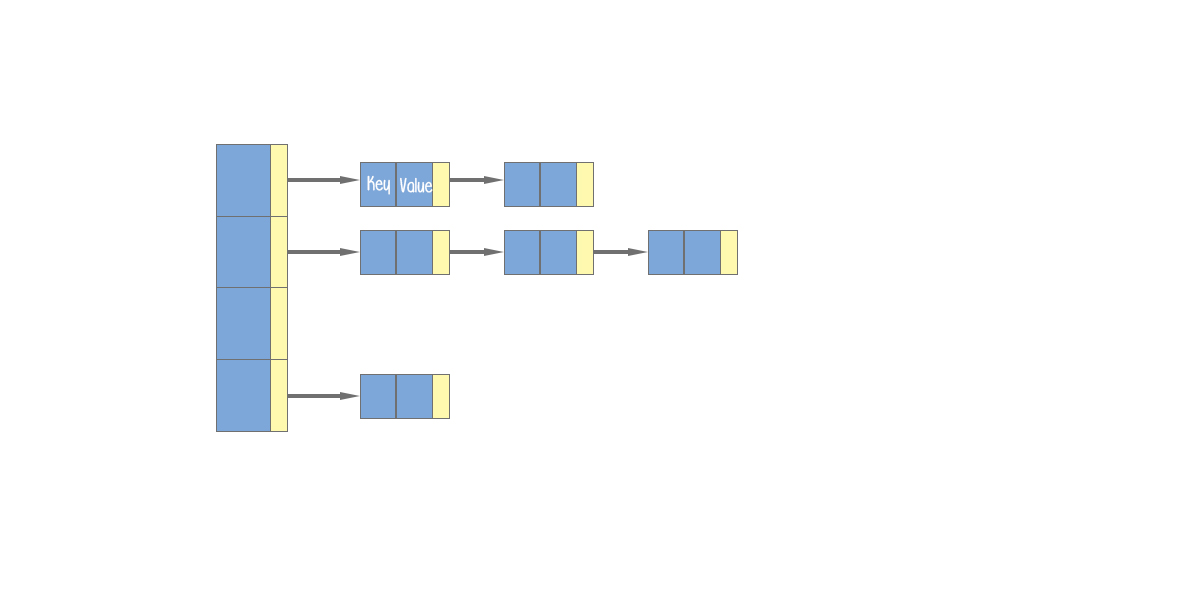### Define classes

The first thing is to define the Entry class. It has two attributes, key and value. Their data type can be anything such as Integer, String or object.

After defining the Entry, we can define HashTable class. It has two attributes, buckets and maxSize. buckets is an array of list of Entry. maxSize is the max length of the buckets.

Here are the reason why we use list of entries, not Entry itself. The hash function will assign each key to a unique bucket. But it is possible that two keys will generate an identical hash value, which causes both keys to point to the same bucket. this is called Collisions. Multiple (key, value) pairs coming to the same bucket form a list in the bucket. Ideally, hashing is O(1) for all insert, delete, and access. But because of collisions, we cannot guarantee the constant time in the worst case.

## Python

### Hashing and hash function

Hashing is a technique to map a range of keys to a range of index of the buckets (aka array). If both ranges are similar, no hash function is necessary. The key values can be used directly as array indices.

But more often the range of the indices is much smaller than the range of the keys. The hash function hashes(converts) a number in a large range into a number in a smaller range. A easy and common hash function is to use the modulo operator(%). The formula is:

hashCode = key % maxSize.

hashCode is the index of buckets. key in the key of the input entry. maxSize is max length of buckets. To distribute hashCode more evenly over the array, maxSize is suggested to be prime number.

Load factor is the ratio of items in a hash table to the array size. A load factor of 1, roughly one third of the cells will be empty, one third will hold one item, and one third will hold two or more items. The load factor can be checked as threshold when to rehashing (resize the buckets in the hash table). In Java Collections, HashMap’s load factor is 0.75.

## Python

### Put in hash table implementation

Put operation is to save a (key, value) pair to the buckets. First it calls hashFunc to get the hashCode, i.e the index of the buckets to access the right bucket. If the bucket’s list is null, create a new list. Then add the new entry of given (key, value) pair to the end of the list.

## Doodle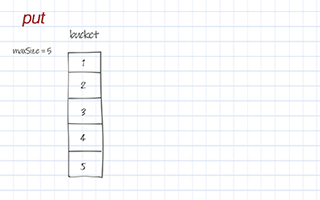### Delete

Delete by key is to delete all entries with that key. The same as put, the first thing is to call hashFunc to get the hashCode to access the right bucket. Then compare the key with each entry’s key in the list. In order to be thread-safe, a new list is created to backup the entries whose key is not the same as the input key. If all entries in the list should be deleted, set the list in this bucket to be null. Otherwise reset the list of this bucket to the new list.

## Doodle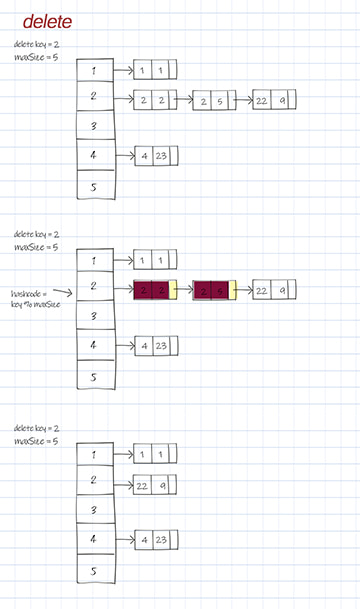### Get

Get operation is to get the value by the key. The same as put, the first thing is to call hashFunc to get the hashCode to access the right bucket. Then compare the key with each entry’s key in the list. Return the first matched entry’s value.

If there is only one item in the list, the time complexity is O(1). If there are many items in the list, access time is O(n) in worst scenario. n is the length of this list. The average time is dependent on load factor. As load factor increases, the length of list grows. So is the access time.

## Doodle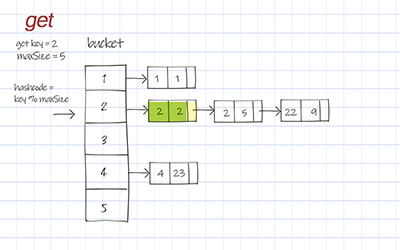### Print

Print is to print all entries in the Hash table. It loops through each bucket and calls a method printList to print elements in the list. If the list is empty, skip it.

## Doodle